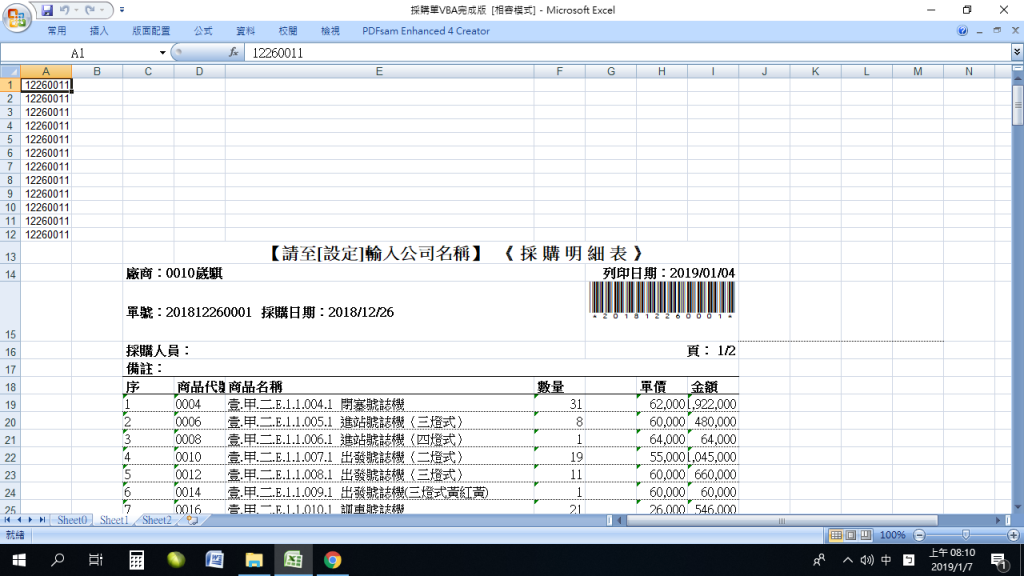0

EXCEL的問題2Range("A1").Select
For C0 = 1 To 500
For i = 1 To 12
If (Mid(Range("C" & C0), 1, 2) = "單號") Then
Range("A" & i) = Mid(Range("C" & C0), 8, 8)
If (Range("A" & i) <> "") Then
Range("A" & i + 1).Select
End If
End If
Next
Next

A2的儲存格是找到單號並擷取的結果

idnoidno iT邦新手 5 級 ‧ 2019-01-07 13:08:22 檢舉

@idnoidno 抱歉

paicheng0111 iT邦研究生 1 級 ‧ 2019-01-07 23:35:04 檢舉

3 個回答

0

Range("A1").Select'起始選取

'從C1到C500尋找單號
For C0 = 1 To 500
'A1到A12(填入單號值、選取)
For i = 1 To 12
'發現單號後，A1到A12儲存格全部填入一樣單號
If (Mid(Range("C" & C0), 1, 2) = "單號") Then
Range("A" & i) = Mid(Range("C" & C0), 8, 8)
'這格有值的話，選取下格
If (Range("A" & i) <> "") Then
Range("A" & i + 1).Select
End If
End If
Next
Next

0

Range("A" & i + 1).Select

0

Dim i as Integer

i = 0
For C0 = 1 To 500
If (Mid(Range("C" & C0), 1, 2) = "單號") Then
Range("A1").Offset(i).Value = Mid(Range("C" & C0), 8, 8)
i = i + 1
End If
Next C0

idnoidno iT邦新手 5 級 ‧ 2019-01-08 11:21:12 檢舉

RANGE的方式建議可改為CELLS，也許比較好也說不定